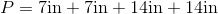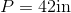# ISEE Middle Level Math : How to find the perimeter of the rectangle

## Example Questions

### Example Question #141 : Quadrilaterals

Find the perimeter of a rectangle with a length of 8cm and a width that is half the length.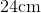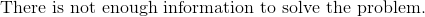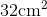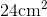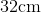Explanation:

To find the perimeter of a rectangle, we will use the following formula: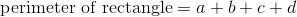where a, b, c, and d are the lengths of the sides of the rectangle.

Now, we know the length of the rectangle is 8cm.  Because it is a rectangle, we know the opposite side is also 8cm.  We also know the width is half the length.  Therefore, the width of the rectangle is 4cm.  Because it is a rectangle, we know the opposite side is also 4cm.  Knowing this, we can substitute into the formula.  We get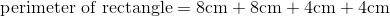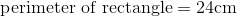### Example Question #142 : Quadrilaterals

Find the perimeter of a rectangle with a width of 4in and a length that is 3 times the width.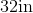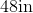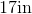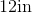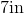Explanation:

To find the perimeter of a rectangle, we will use the following formula:where a, b, c, and d are the lengths of the sides of the rectangle.

Now, we know the width of the rectangle is 4in.  Because it is a rectangle, we know the opposite side is also 4in.

We know the length is 3 times the width.  Therefore, the length is 12in.  Because it is a rectangle, we know the opposite side is also 12in.

Knowing all of this, we can substitute into the formula.  We get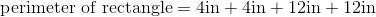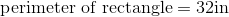### Example Question #81 : Rectangles

Find the perimeter of a rectangle with a width of 8cm and a length that is three times the width.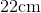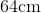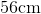Explanation:

To find the perimeter of a rectangle, we will use the following formula:where a, b, c, and d are the lengths of the sides of the rectangle.

Now, we know the width of the rectangle is 8cm.  Because it is a rectangle, we know the opposite side is also 8cm.  We also know the length is three times the width.  Therefore, the length is 24cm.  Because it is a rectangle, we know the opposite side is also 24cm.

Knowing all of this, we can substitute into the formula.  We get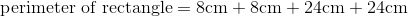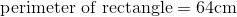### Example Question #82 : Rectangles

Use the following image to answer the question: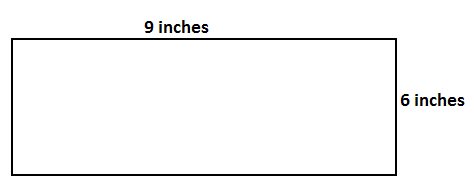Find the perimeter of the rectangle.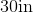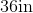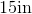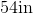Explanation:

To find the perimeter of a rectangle, we will use the following formula:where a, b, c, and d are the lengths of the sides of the rectangle.

Now, let's look at the rectangle.We can see the length is 9 inches.  Because it is a rectangle, the opposite side is also 9 inches.  We can see the width is 6 inches.  Because it is a rectangle, the opposite side is also 6 inches.

Knowing this, we can substitute into the formula.  We get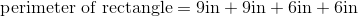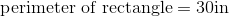### Example Question #83 : Rectangles

Find the perimeter of a rectangle with a length of 8cm and a width that is half the length.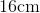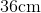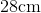Explanation:

To find the perimeter of a rectangle, we will use the following formula:where a, b, c, and d are the lengths of the sides of the rectangle.

Now, we know the length of the rectangle is 8cm.  Because it is a rectangle, we know the opposite side is also 8cm.  We know the width of the rectangle is half the length.  Therefore, the width is 4cm.  Because it is a rectangle, the opposite side is also 4cm.

Knowing this, we can substitute into the formula.  We get### Example Question #84 : Rectangles

Find the perimeter of a rectangle with a length of 7in and a width of 4in.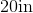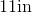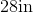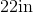Explanation:

To find the perimeter of a rectangle, we will use the following formula:where a, b, c, and d are the lengths of the sides of the rectangle.

Now, we know the length of the rectangle is 7in.  Because it is a rectangle, the opposite side is also 7in.

We also know the width of the rectangle is 4in.  Because it is a rectangle, the opposite side is also 4in.

Knowing this, we can substitute into the formula.  We get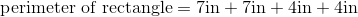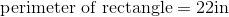### Example Question #85 : Rectangles

Find the perimeter of a rectangle with a width of 4in and a length that is three times the width.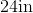Explanation:

To find the perimeter of a rectangle, we will use the following formula:where a, b, c, and d are the lengths of the sides of the rectangle.

Now, we know the width of the rectangle is 4in.  Because it is a rectangle, we know the opposite side is also 4in.

We also know the length is three times the width.  Therefore, the length is 12in.  Because it is a rectangle, the opposite side is also 12in.

Knowing this, we can substitute into the formula.  We get### Example Question #86 : Rectangles

Find the perimeter of a rectangle with a length of 12cm and a width that is a quarter of the length.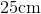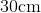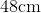Explanation:

To find the perimeter of a rectangle, we will use the following formula:Where a, b, c, and d are the lengths of the sides of the rectangle.

Now, we know the length of the rectangle is 12cm.  Because it is a rectangle, the opposite side is also 12cm.  We also know the width of the rectangle is a quarter of the length.  To find the width, we will divide 12 by 4.  Therefore, the width is 3cm.  Because it is a rectangle, the opposite side is also 3cm.

Knowing this, we can substitute into the formula.  We get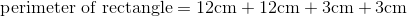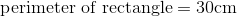### Example Question #87 : Rectangles

Find the perimeter of a rectangle that has a width of 7in and a length that is three times the width.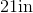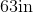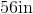Explanation:

To find the perimeter of a rectangle, we will use the following formula:Where a, b, c, and d are the lengths of the sides of the rectangle.

Now, we know the width of the rectangle is 7in.  Because it is a rectangle, the opposite side is also 7in.  We also know the length of the rectangle is three times the width.  Therefore, the length is 21in.  Because it is a rectangle, the opposite side is also 21in.

Knowing this, we can substitute into the formula.  We get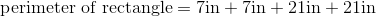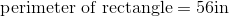### Example Question #88 : Rectangles

Find the perimeter of a rectangle with a width of 7in and a length that is two times the width.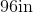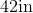Explanation:

To find the perimeter of a rectangle, we will use the following formula: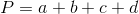Where a, b, c, and d are the lengths of the sides of the rectangle.

Now, we know the width of the rectangle is 7in.  Because it is a rectangle, the opposite side is also 7in.

We know the length is two times the width.  Therefore, the length is 14in.  Because it is a rectangle, the opposite side is also 14in.

Knowing this, we can substitute into the formula.  We get